Intermediate Algebra

# 11.1Distance and Midpoint Formulas; Circles

Intermediate Algebra11.1 Distance and Midpoint Formulas; Circles

### Learning Objectives

By the end of this section, you will be able to:
• Use the Distance Formula
• Use the Midpoint Formula
• Write the equation of a circle in standard form
• Graph a circle

### Be Prepared 11.1

Before you get started, take this readiness quiz.

1. Find the length of the hypotenuse of a right triangle whose legs are 12 and 16 inches.
If you missed this problem, review Example 2.34.
2. Factor: $x2−18x+81.x2−18x+81.$
If you missed this problem, review Example 6.24.
3. Solve by completing the square: $x2−12x−12=0.x2−12x−12=0.$
If you missed this problem, review Example 9.22.

In this chapter we will be looking at the conic sections, usually called the conics, and their properties. The conics are curves that result from a plane intersecting a double cone—two cones placed point-to-point. Each half of a double cone is called a nappe.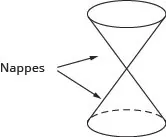There are four conics—the circle, parabola, ellipse, and hyperbola. The next figure shows how the plane intersecting the double cone results in each curve.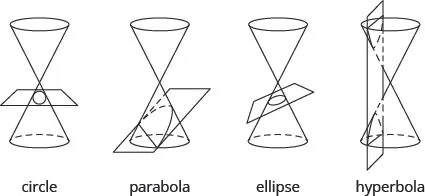Each of the curves has many applications that affect your daily life, from your cell phone to acoustics and navigation systems. In this section we will look at the properties of a circle.

### Use the Distance Formula

We have used the Pythagorean Theorem to find the lengths of the sides of a right triangle. Here we will use this theorem again to find distances on the rectangular coordinate system. By finding distance on the rectangular coordinate system, we can make a connection between the geometry of a conic and algebra—which opens up a world of opportunities for application.

Our first step is to develop a formula to find distances between points on the rectangular coordinate system. We will plot the points and create a right triangle much as we did when we found slope in Graphs and Functions. We then take it one step further and use the Pythagorean Theorem to find the length of the hypotenuse of the triangle—which is the distance between the points.

### Example 11.1

Use the rectangular coordinate system to find the distance between the points $(6,4)(6,4)$ and $(2,1).(2,1).$

### Try It 11.1

Use the rectangular coordinate system to find the distance between the points $(6,1)(6,1)$ and $(2,−2).(2,−2).$

### Try It 11.2

Use the rectangular coordinate system to find the distance between the points $(5,3)(5,3)$ and $(−3,−3).(−3,−3).$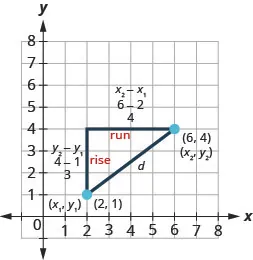The method we used in the last example leads us to the formula to find the distance between the two points $(x1,y1)(x1,y1)$ and $(x2,y2).(x2,y2).$

When we found the length of the horizontal leg we subtracted $6−26−2$ which is $x2−x1.x2−x1.$

When we found the length of the vertical leg we subtracted $4−14−1$ which is $y2−y1.y2−y1.$

If the triangle had been in a different position, we may have subtracted $x1−x2x1−x2$ or $y1−y2.y1−y2.$ The expressions $x2−x1x2−x1$ and $x1−x2x1−x2$ vary only in the sign of the resulting number. To get the positive value-since distance is positive- we can use absolute value. So to generalize we will say $|x2−x1||x2−x1|$ and $|y2−y1|.|y2−y1|.$

In the Pythagorean Theorem, we substitute the general expressions $|x2−x1||x2−x1|$ and $|y2−y1||y2−y1|$ rather than the numbers.

$a2+b2=c2 Substitute in the values.(|x2−x1|)2+(|y2−y1|)2=d2 Squaring the expressions makes thempositive, so we eliminate the absolute valuebars.(x2−x1)2+(y2−y1)2=d2 Use the Square Root Property.d=±(x2−x1)2+(y2−y1)2 Distance is positive, so eliminate the negativevalue.d=(x2−x1)2+(y2−y1)2 a2+b2=c2 Substitute in the values.(|x2−x1|)2+(|y2−y1|)2=d2 Squaring the expressions makes thempositive, so we eliminate the absolute valuebars.(x2−x1)2+(y2−y1)2=d2 Use the Square Root Property.d=±(x2−x1)2+(y2−y1)2 Distance is positive, so eliminate the negativevalue.d=(x2−x1)2+(y2−y1)2$

This is the Distance Formula we use to find the distance d between the two points $(x1,y1)(x1,y1)$ and $(x2,y2).(x2,y2).$

### Distance Formula

The distance d between the two points $(x1,y1)(x1,y1)$ and $(x2,y2)(x2,y2)$ is

$d=(x2−x1)2+(y2−y1)2d=(x2−x1)2+(y2−y1)2$

### Example 11.2

Use the Distance Formula to find the distance between the points $(−5,−3)(−5,−3)$ and $(7,2).(7,2).$

### Try It 11.3

Use the Distance Formula to find the distance between the points $(−4,−5)(−4,−5)$ and $(5,7).(5,7).$

### Try It 11.4

Use the Distance Formula to find the distance between the points $(−2,−5)(−2,−5)$ and $(−14,−10).(−14,−10).$

### Example 11.3

Use the Distance Formula to find the distance between the points $(10,−4)(10,−4)$ and $(−1,5).(−1,5).$ Write the answer in exact form and then find the decimal approximation, rounded to the nearest tenth if needed.

### Try It 11.5

Use the Distance Formula to find the distance between the points $(−4,−5)(−4,−5)$ and $(3,4).(3,4).$ Write the answer in exact form and then find the decimal approximation, rounded to the nearest tenth if needed.

### Try It 11.6

Use the Distance Formula to find the distance between the points $(−2,−5)(−2,−5)$ and $(−3,−4).(−3,−4).$ Write the answer in exact form and then find the decimal approximation, rounded to the nearest tenth if needed.

### Use the Midpoint Formula

It is often useful to be able to find the midpoint of a segment. For example, if you have the endpoints of the diameter of a circle, you may want to find the center of the circle which is the midpoint of the diameter. To find the midpoint of a line segment, we find the average of the x-coordinates and the average of the y-coordinates of the endpoints.

### Midpoint Formula

The midpoint of the line segment whose endpoints are the two points $(x1,y1)(x1,y1)$ and $(x2,y2)(x2,y2)$ is

$(x1+x22,y1+y22)(x1+x22,y1+y22)$

To find the midpoint of a line segment, we find the average of the x-coordinates and the average of the y-coordinates of the endpoints.

### Example 11.4

Use the Midpoint Formula to find the midpoint of the line segments whose endpoints are $(−5,−4)(−5,−4)$ and $(7,2).(7,2).$ Plot the endpoints and the midpoint on a rectangular coordinate system.

### Try It 11.7

Use the Midpoint Formula to find the midpoint of the line segments whose endpoints are $(−3,−5)(−3,−5)$ and $(5,7).(5,7).$ Plot the endpoints and the midpoint on a rectangular coordinate system.

### Try It 11.8

Use the Midpoint Formula to find the midpoint of the line segments whose endpoints are $(−2,−5)(−2,−5)$ and $(6,−1).(6,−1).$ Plot the endpoints and the midpoint on a rectangular coordinate system.

Both the Distance Formula and the Midpoint Formula depend on two points, $(x1,y1)(x1,y1)$ and $(x2,y2).(x2,y2).$ It is easy to confuse which formula requires addition and which subtraction of the coordinates. If we remember where the formulas come from, is may be easier to remember the formulas.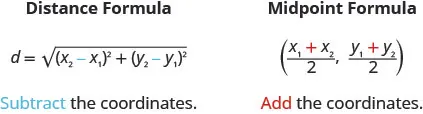### Write the Equation of a Circle in Standard Form

As we mentioned, our goal is to connect the geometry of a conic with algebra. By using the coordinate plane, we are able to do this easily.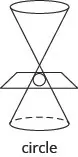We define a circle as all points in a plane that are a fixed distance from a given point in the plane. The given point is called the center, $(h,k),(h,k),$ and the fixed distance is called the radius, r, of the circle.

### Circle

A circle is all points in a plane that are a fixed distance from a given point in the plane. The given point is called the center, $(h,k),(h,k),$ and the fixed distance is called the radius, r, of the circle.

 We look at a circle in the rectangular coordinate system.The radius is the distance from the center, $(h,k),(h,k),$ to apoint on the circle, $(x,y).(x,y).$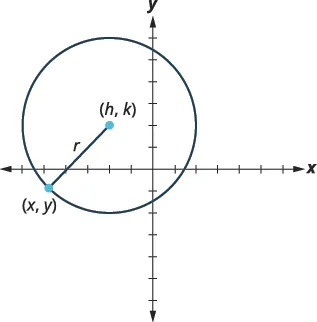To derive the equation of a circle, we can use thedistance formula with the points $(h,k),(h,k),$ $(x,y)(x,y)$ and thedistance, r. $d=(x2−x1)2+(y2−y1)2d=(x2−x1)2+(y2−y1)2$ Substitute the values. $r=(x−h)2+(y−k)2r=(x−h)2+(y−k)2$ Square both sides. $r2=(x−h)2+(y−k)2r2=(x−h)2+(y−k)2$

This is the standard form of the equation of a circle with center, $(h,k),(h,k),$ and radius, r.

### Standard Form of the Equation a Circle

The standard form of the equation of a circle with center, $(h,k),(h,k),$ and radius, r, is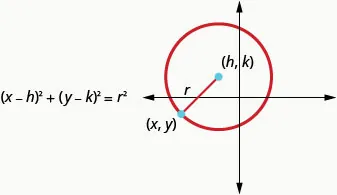### Example 11.5

Write the standard form of the equation of the circle with radius 3 and center $(0,0).(0,0).$

### Try It 11.9

Write the standard form of the equation of the circle with a radius of 6 and center $(0,0).(0,0).$

### Try It 11.10

Write the standard form of the equation of the circle with a radius of 8 and center $(0,0).(0,0).$

In the last example, the center was $(0,0).(0,0).$ Notice what happened to the equation. Whenever the center is $(0,0),(0,0),$ the standard form becomes $x2+y2=r2.x2+y2=r2.$

### Example 11.6

Write the standard form of the equation of the circle with radius 2 and center $(−1,3).(−1,3).$

### Try It 11.11

Write the standard form of the equation of the circle with a radius of 7 and center $(2,−4).(2,−4).$

### Try It 11.12

Write the standard form of the equation of the circle with a radius of 9 and center $(−3,−5).(−3,−5).$

In the next example, the radius is not given. To calculate the radius, we use the Distance Formula with the two given points.

### Example 11.7

Write the standard form of the equation of the circle with center $(2,4)(2,4)$ that also contains the point $(−2,1).(−2,1).$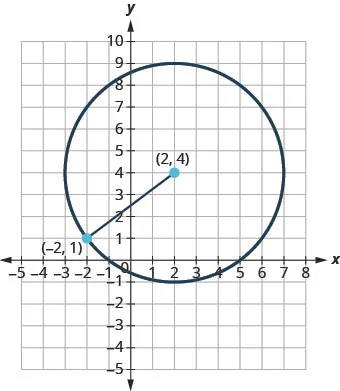### Try It 11.13

Write the standard form of the equation of the circle with center $(2,1)(2,1)$ that also contains the point $(−2,−2).(−2,−2).$

### Try It 11.14

Write the standard form of the equation of the circle with center $(7,1)(7,1)$ that also contains the point $(−1,−5).(−1,−5).$

### Graph a Circle

Any equation of the form $(x−h)2+(y−k)2=r2(x−h)2+(y−k)2=r2$ is the standard form of the equation of a circle with center, $(h,k),(h,k),$ and radius, r. We can then graph the circle on a rectangular coordinate system.

Note that the standard form calls for subtraction from x and y. In the next example, the equation has $x+2,x+2,$ so we need to rewrite the addition as subtraction of a negative.

### Example 11.8

Find the center and radius, then graph the circle: $(x+2)2+(y−1)2=9.(x+2)2+(y−1)2=9.$

### Try It 11.15

Find the center and radius, then graph the circle: $(x−3)2+(y+4)2=4.(x−3)2+(y+4)2=4.$

### Try It 11.16

Find the center and radius, then graph the circle: $(x−3)2+(y−1)2=16.(x−3)2+(y−1)2=16.$

To find the center and radius, we must write the equation in standard form. In the next example, we must first get the coefficient of $x2,y2x2,y2$ to be one.

### Example 11.9

Find the center and radius and then graph the circle, $4x2+4y2=64.4x2+4y2=64.$

### Try It 11.17

Find the center and radius, then graph the circle: $3x2+3y2=273x2+3y2=27$

### Try It 11.18

Find the center and radius, then graph the circle: $5x2+5y2=1255x2+5y2=125$

If we expand the equation from Example 11.8, $(x+2)2+(y−1)2=9,(x+2)2+(y−1)2=9,$ the equation of the circle looks very different.

$(x+2)2+(y−1)2=9 Square the binomials.x2+4x+4+y2−2y+1=9 Arrange the terms in descending degree order,and get zero on the rightx2+y2+4x−2y−4=0 (x+2)2+(y−1)2=9 Square the binomials.x2+4x+4+y2−2y+1=9 Arrange the terms in descending degree order,and get zero on the rightx2+y2+4x−2y−4=0$

This form of the equation is called the general form of the equation of the circle.

### General Form of the Equation of a Circle

The general form of the equation of a circle is

$x2+y2+ax+by+c=0x2+y2+ax+by+c=0$

If we are given an equation in general form, we can change it to standard form by completing the squares in both x and y. Then we can graph the circle using its center and radius.

### Example 11.10

Find the center and radius, then graph the circle: $x2+y2−4x−6y+4=0.x2+y2−4x−6y+4=0.$

### Try It 11.19

Find the center and radius, then graph the circle: $x2+y2−6x−8y+9=0.x2+y2−6x−8y+9=0.$

### Try It 11.20

Find the center and radius, then graph the circle: $x2+y2+6x−2y+1=0.x2+y2+6x−2y+1=0.$

In the next example, there is a y-term and a $y2y2$-term. But notice that there is no x-term, only an $x2x2$-term. We have seen this before and know that it means h is 0. We will need to complete the square for the y terms, but not for the x terms.

### Example 11.11

Find the center and radius, then graph the circle: $x2+y2+8y=0.x2+y2+8y=0.$

### Try It 11.21

Find the center and radius, then graph the circle: $x2+y2−2x−3=0.x2+y2−2x−3=0.$

### Try It 11.22

Find the center and radius, then graph the circle: $x2+y2−12y+11=0.x2+y2−12y+11=0.$

### Media

Access these online resources for additional instructions and practice with using the distance and midpoint formulas, and graphing circles.

### Section 11.1 Exercises

#### Practice Makes Perfect

Use the Distance Formula

In the following exercises, find the distance between the points. Write the answer in exact form and then find the decimal approximation, rounded to the nearest tenth if needed.

1.

$(2,0)(2,0)$ and $(5,4)(5,4)$

2.

$(−4,−3)(−4,−3)$ and $(2,5)(2,5)$

3.

$(−4,−3)(−4,−3)$ and $(8,2)(8,2)$

4.

$(−7,−3)(−7,−3)$ and $(8,5)(8,5)$

5.

$(−1,4)(−1,4)$ and $(2,0)(2,0)$

6.

$(−1,3)(−1,3)$ and $(5,−5)(5,−5)$

7.

$(1,−4)(1,−4)$ and $(6,8)(6,8)$

8.

$(−8,−2)(−8,−2)$ and $(7,6)(7,6)$

9.

$(−3,−5)(−3,−5)$ and $(0,1)(0,1)$

10.

$(−1,−2)(−1,−2)$ and $(−3,4)(−3,4)$

11.

$(3,−1)(3,−1)$ and $(1,7)(1,7)$

12.

$(−4,−5)(−4,−5)$ and $(7,4)(7,4)$

Use the Midpoint Formula

In the following exercises, find the midpoint of the line segments whose endpoints are given and plot the endpoints and the midpoint on a rectangular coordinate system.

13.

$(0,−5)(0,−5)$ and $(4,−3)(4,−3)$

14.

$(−2,−6)(−2,−6)$ and $(6,−2)(6,−2)$

15.

$(3,−1)(3,−1)$ and $(4,−2)(4,−2)$

16.

$(−3,−3)(−3,−3)$ and $(6,−1)(6,−1)$

Write the Equation of a Circle in Standard Form

In the following exercises, write the standard form of the equation of the circle with the given radius and center $(0,0).(0,0).$

17.

18.

19.

Radius: $22$

20.

Radius: $55$

In the following exercises, write the standard form of the equation of the circle with the given radius and center

21.

Radius: 1, center: $(3,5)(3,5)$

22.

Radius: 10, center: $(−2,6)(−2,6)$

23.

Radius: $2.5,2.5,$ center: $(1.5,−3.5)(1.5,−3.5)$

24.

Radius: $1.5,1.5,$ center: $(−5.5,−6.5)(−5.5,−6.5)$

For the following exercises, write the standard form of the equation of the circle with the given center with point on the circle.

25.

Center $(3,−2)(3,−2)$ with point $(3,6)(3,6)$

26.

Center $(6,−6)(6,−6)$ with point $(2,−3)(2,−3)$

27.

Center $(4,4)(4,4)$ with point $(2,2)(2,2)$

28.

Center $(−5,6)(−5,6)$ with point $(−2,3)(−2,3)$

Graph a Circle

In the following exercises, find the center and radius, then graph each circle.

29.

$( x + 5 ) 2 + ( y + 3 ) 2 = 1 ( x + 5 ) 2 + ( y + 3 ) 2 = 1$

30.

$( x − 2 ) 2 + ( y − 3 ) 2 = 9 ( x − 2 ) 2 + ( y − 3 ) 2 = 9$

31.

$( x − 4 ) 2 + ( y + 2 ) 2 = 16 ( x − 4 ) 2 + ( y + 2 ) 2 = 16$

32.

$( x + 2 ) 2 + ( y − 5 ) 2 = 4 ( x + 2 ) 2 + ( y − 5 ) 2 = 4$

33.

$x 2 + ( y + 2 ) 2 = 25 x 2 + ( y + 2 ) 2 = 25$

34.

$( x − 1 ) 2 + y 2 = 36 ( x − 1 ) 2 + y 2 = 36$

35.

$( x − 1.5 ) 2 + ( y + 2.5 ) 2 = 0.25 ( x − 1.5 ) 2 + ( y + 2.5 ) 2 = 0.25$

36.

$( x − 1 ) 2 + ( y − 3 ) 2 = 9 4 ( x − 1 ) 2 + ( y − 3 ) 2 = 9 4$

37.

$x 2 + y 2 = 64 x 2 + y 2 = 64$

38.

$x 2 + y 2 = 49 x 2 + y 2 = 49$

39.

$2 x 2 + 2 y 2 = 8 2 x 2 + 2 y 2 = 8$

40.

$6 x 2 + 6 y 2 = 216 6 x 2 + 6 y 2 = 216$

In the following exercises, identify the center and radius and graph.

41.

$x 2 + y 2 + 2 x + 6 y + 9 = 0 x 2 + y 2 + 2 x + 6 y + 9 = 0$

42.

$x 2 + y 2 − 6 x − 8 y = 0 x 2 + y 2 − 6 x − 8 y = 0$

43.

$x 2 + y 2 − 4 x + 10 y − 7 = 0 x 2 + y 2 − 4 x + 10 y − 7 = 0$

44.

$x 2 + y 2 + 12 x − 14 y + 21 = 0 x 2 + y 2 + 12 x − 14 y + 21 = 0$

45.

$x 2 + y 2 + 6 y + 5 = 0 x 2 + y 2 + 6 y + 5 = 0$

46.

$x 2 + y 2 − 10 y = 0 x 2 + y 2 − 10 y = 0$

47.

$x 2 + y 2 + 4 x = 0 x 2 + y 2 + 4 x = 0$

48.

$x 2 + y 2 − 14 x + 13 = 0 x 2 + y 2 − 14 x + 13 = 0$

#### Writing Exercises

49.

Explain the relationship between the distance formula and the equation of a circle.

50.

Is a circle a function? Explain why or why not.

51.

In your own words, state the definition of a circle.

52.

In your own words, explain the steps you would take to change the general form of the equation of a circle to the standard form.

#### Self Check

After completing the exercises, use this checklist to evaluate your mastery of the objectives of this section.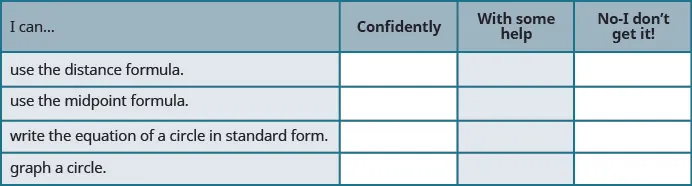If most of your checks were:

…confidently. Congratulations! You have achieved the objectives in this section. Reflect on the study skills you used so that you can continue to use them. What did you do to become confident of your ability to do these things? Be specific.

…with some help. This must be addressed quickly because topics you do not master become potholes in your road to success. In math every topic builds upon previous work. It is important to make sure you have a strong foundation before you move on. Who can you ask for help? Your fellow classmates and instructor are good resources. Is there a place on campus where math tutors are available? Can your study skills be improved?

…no - I don’t get it! This is a warning sign and you must not ignore it. You should get help right away or you will quickly be overwhelmed. See your instructor as soon as you can to discuss your situation. Together you can come up with a plan to get you the help you need.

Order a print copy

As an Amazon Associate we earn from qualifying purchases.